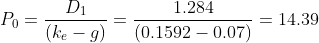Scan QR code or get instant email to install app

Question:

# The value per share is closest to:

A \$14.39.
explanation

1212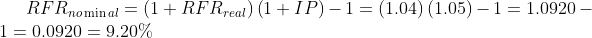Using the CAPM, the required rate of return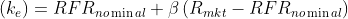= 9.20% + 1.4(14.0% − 9.2%) = 9.20% +6.72% = 15.92%
The retention ratio (RR) = 1 − dividend payout ratio = 1 − 0.30 = 0.70
The growth rate (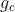) = (RR)(ROE) = (0.70)(10%) = 7.00%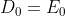(dividend payout) = 4.00(0.30) = 1.20
Next year's dividend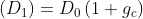= 1.20(1 + 0.07) = 1.284